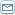# Real Estate Math - Do You Know These Simple Formulas?

 By: Steven GillmanHow much real estate math do you need to know if you are investing in real estate? There are computers and calculators for calculating interest rates or amortizing loans. What you need to know is a few simple formulas for determining if a property is a good investment or not.The Real Estate Math You Don't NeedThe gross rent multiplier is one formula you don't need. I bring it up because people are sometimes still using it, and there are better ways to estimate value. A gross rent multiplier is a crude way to put a value on a property. You decide that properties are worth 10 times annual rent or less, for example, and simply multiply the gross annual rent a building collects by ten to get your value.There are obvious problems with this formula. You need to constantly change it to reflect interest rates, because a property might be profitable at 12 times rent when interest rates are low, but a money loser at eight times rent if the financing is expensive. Also, there are just plain different expenses for different properties, especially when some include utilities in the rent, for example. Gross rent doesn't say much about the factor that makes a property valuable: the net income.Real Estate Math You NeedRental properties are bought for the income they produce, so this is what your real estate valuation should be based on. That is why your real estate math education needs to start with the how to use a capitalization rate, or "cap rate" to determine value. A cap rate is the rate of return expected by investors in a given area, or the rate of return on a property at a given price. An example might make this clear. Take the gross income of a property and subtract all expenses, but not the loan payments. If the gross income is \$76,000 per year, and the expenses are \$32,000, you have net income before debt-service of \$44,000. Now, to arrive at an estimate of value, you simply apply the capitalization rate to this figure.If the normal capitalization rate is .10 (ask a real estate professional what is normal in your area), meaning investors expect a 10% return on the value of their investment, you would  divide the net income of \$44,000 by .10. You get \$440,000 - the estimated value of the building. If the common rate is .08, meaning investors in the area expect only an 8% return, the value would be \$550,000.Simple Real Estate MathEstimated value equals net income before debt-service divided by cap rate - this really is simple real estate math, but the tough part is getting accurate income figures. Is the seller is showing you ALL the normal expenses, and not exaggerating income? If he stopped repairing things for a year, and is showing "projected" rents, instead of actual rents collected, the income figure could be \$15,000 too high. That would mean you would estimate the value at \$187,000 more (.08 cap rate).Besides verifying the figures, smart investors sometimes separate out income from vending machines and laundry machines. Suppose these sources provide \$6,000 of the income. That would add \$75,000 to the appraised value (.08 cap rate). Instead, you can do the appraisal without this income included, then add back the replacement cost of the machines (probably much less than \$75,000).No real estate formula is perfect, and all are only as good as the figures you plug into them. Used carefully, though, real estate appraisal using capitalization rates is the most accurate method for estimating the value of income properties. For putting a value on a single family home, you need another approach. Yes this means more real estate math to learn, but we'll save that for another time.